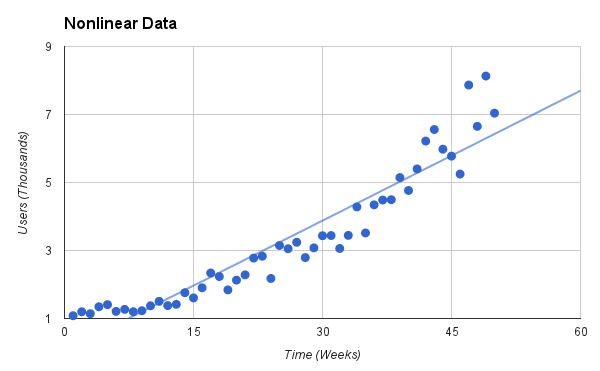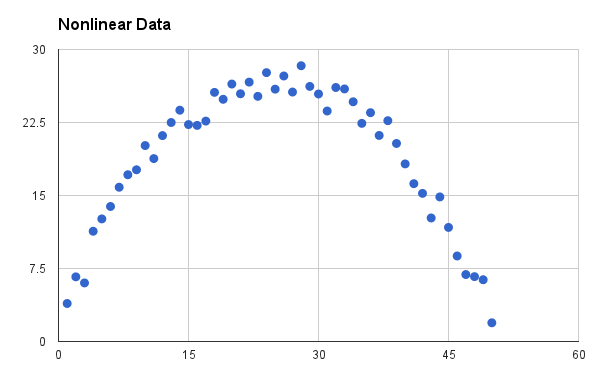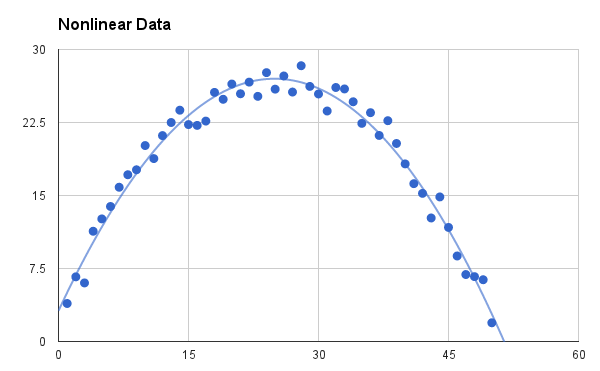# Everyday Statistics for Programmers: Nonlinear Regression

Last week I talked about how to figure out if two variables in your data set are correlated, and the week before I talked about fitting a trend line to your data. What happens if you know your data is correlated, but the relationship doesn't look linear? Is there anything that can be done to estimate the trend in the data if it's not linear? Yes, depending on what the data looks like, we can either transform the data to make it linear, or do a polynomial regression on the data to fit a polynomial equation to it.

We'll take a closer look at data transformations, and then briefly cover polynomial regression. The idea with data transformations is to somehow make your data linear. There are a few data trends that this will work for, and luckily, these types of trends cover most of the nonlinear data that you're ever likely to see. These trends fall into the categories of exponential, power, logarithmic, and reciprocal relationships.

The exponential relationship is probably the most common of these, so lets go through an example of how to transform a set of data that exhibits an exponential trend. Say you've started a new website, and you're measuring the number of active users on your site each week. Your usage data might look something like this:The linear trend line is there to show that the data is not really linear. It's kind of linear, but in the middle of the scatter plot the data bows below the line and at the end of the plot it looks to be taking off above the line. One would assume that to get a better idea of what might happen in the near future, using a linear trend line will underestimate the site's performance. To get a better idea, we can use linear regression, but first we want to transform the data to make it more linear. In this case that means taking the logarithm of the y-values of the data, which produces the following scatter plot of the transformed data:The transformed data definitely looks more linear, as the trend line running right through the scatter plot shows. You can do a linear regression on it in exactly the same way as any other linear regression, ending up with the m (slope) and b (offset) coefficients. However, we want the coefficients for the original exponential relationship, which is of the form

y = α*exp(ß*x)

Since we took the logarithm of y, the form of the linear equation that we found the coefficients for is

ln(y) = ln(α) + ß*x

This looks just like the linear equation y = m*x + b, so we can conclude that α = exp(b) and ß = m. Now that we have the coefficients of the exponential trend line, we can plot it against the original data like so:Pretty slick. The exponential trend line fits the data much better, and we can merrily be on our way, extrapolating future site growth (but not too far in the future, of course). One thing to be careful of, though. Notice how the data points get more spread out later in time? That's characteristic of many exponential trends, but not all. If the data points look evenly spread out over the entire range of the data (in statistical terms this would be stated as constant variance over the range), then transforming the data will cause the smaller values to be more heavily weighted in the linear regression. A more appropriate analysis to do in this case, if you need the additional accuracy, would be a true nonlinear regression using a nonlinear solver.

A similar procedure to the logarithmic transform for exponential trends works for a power relationship of the form

y = α*x^ß

The difference is that the logarithm of the x-values must also be calculated to give the data a linear form of

ln(y) = ln(α) + ß*ln(x)

The original coefficients are found the same way as they were for the exponential relationship, with α = exp(b) and ß = m. That wasn't too difficult; just a slight tweak was needed to the data transformation.

The last two forms of logarithmic and reciprocal relationships are easier to calculate because only the x-values need to be transformed with a ln(x) and a 1/x operation, respectively. The linear coefficients are the same between the transformed and original data, so once the linear regression is done on the transformed data, the work is pretty much done.

That's all well and good as far as nonlinear regression goes, but what happens if your data looks like this:This data doesn't fit any of the relationships discussed so far. It's an example of a quadratic relationship that also shows up fairly often in different types of data, especially economic data. When you see data like this, you can assume that there is some kind of tradeoff involved, and you probably want to optimize for the area on the scatter plot where the data peaks. To figure out exactly where that peak is, you need to find an equation for the trend line, and to do that, you need polynomial regression.

Before we get into polynomial regression, also note that data with a quadratic relationship doesn't have to look like the scatter plot above. It can also be flipped and shifted so that it looks more like an exponential relationship. The data would have a growth characteristic in this case, instead of a trade-off characteristic. It's often hard to tell the difference between quadratic and exponential growth, especially if the data doesn't span enough time. A trend line might show a misfit if the data increases much faster than a quadratic function. In that case, the exponential fit is definitely the way to go. Otherwise, you'll have to resort to your knowledge of the domain and use your judgement as to which relationship better describes the data.

Cubic relationships can also show up in data from time to time, but they are much more rare. Having data with polynomial relationships larger than third order are more of a warning sign than anything. If you're trying to fit a tenth-order polynomial to your data, you may want to rethink your analysis or question your data. You may be moving out of the realm of statistics and into the realm of signal processing.

Getting back to the problem at hand, how do we find the best fit quadratic curve for the data set shown above? Basically, you can construct a linear equation with matrices and solve for the coefficients. That actually makes polynomial regression a form of linear regression, but it's done on nonlinear data. The math is more involved than we'll get into here, but there is a nice implementation of the algorithm in Rubythat I found on a great programming algorithm website, called rosettacode.org. Here's the code adapted to the Statistics module I've been building:

``````
require 'matrix'

module Statistics

def self.polyfit(x, y, degree)

x_data = x.map { |xi| (0..degree).map { |pow| (xi**pow).to_f } }

mx = Matrix[*x_data]

my = Matrix.column_vector(y)

((mx.t * mx).inv * mx.t * my).t.to_a

end

end
``````

The first three lines of the polyfit() method are building up the X and Y matrices, and then the last line of the method does the actual calculation to produce the coefficients. If you call this method with the (x,y) data from the scatter plot above and set the degree to 2, you'll get the three coefficients out for the equation:

y = a*x^2 + b*x + c

If you then plot this quadratic equation as the trend line for the scatter plot, you get a nice fit of the data:That wraps up the everyday ways of doing regression on nonlinear data. Doing nonlinear regression with transformations on the data or using a polynomial regression should cover the vast majority of nonlinear data that you would come across, and the analysis is fairly straightforward once you decide on which type of curve to fit.

I hope you've enjoyed this miniseries on statistics, and that it proves useful for your everyday data analysis. Happy estimating!